# Subjective Question 1 Kinetics A Increase the rate

• Slides: 54Subjective Question # 1 Kinetics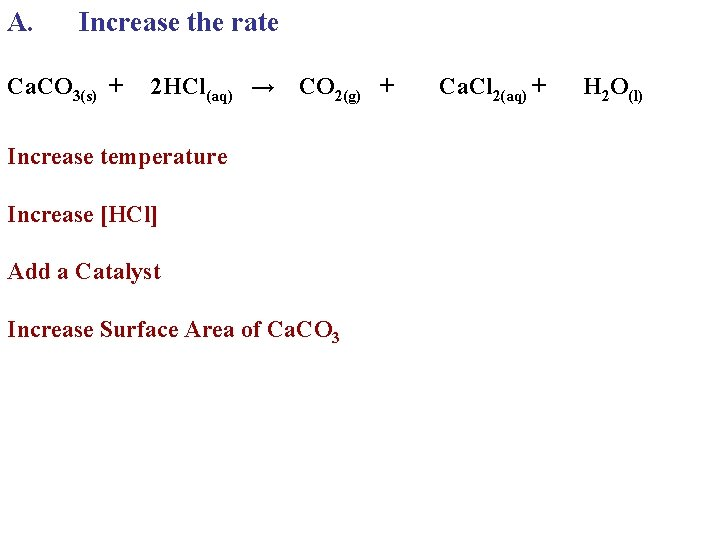A. Increase the rate Ca. CO 3(s) + 2 HCl(aq) → CO 2(g) + Increase temperature Increase [HCl] Add a Catalyst Increase Surface Area of Ca. CO 3 Ca. Cl 2(aq) + H 2 O(l)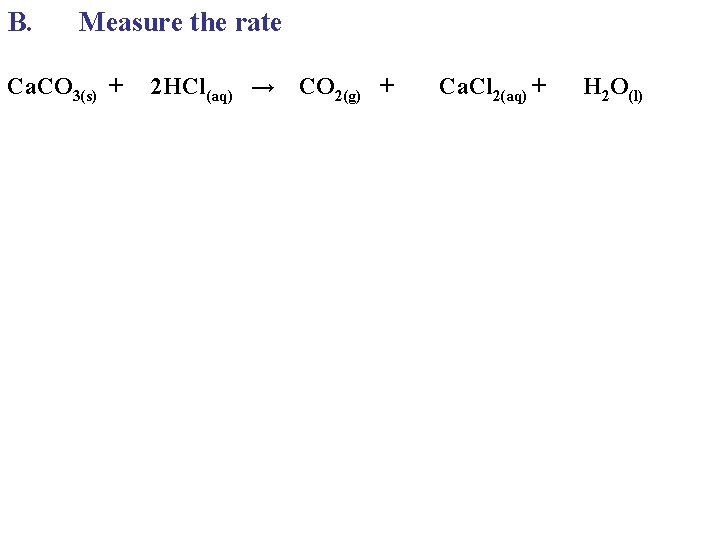B. Measure the rate Ca. CO 3(s) + 2 HCl(aq) → CO 2(g) + Ca. Cl 2(aq) + H 2 O(l)B. Measure the rate Ca. CO 3(s) + 2 HCl(aq) → CO 2(g) + mass Ca. Cl 2(aq) + H 2 O(l)B. Measure the rate Ca. CO 3(s) + 2 HCl(aq) → CO 2(g) + Ca. Cl 2(aq) + mass [HCl] H 2 O(l)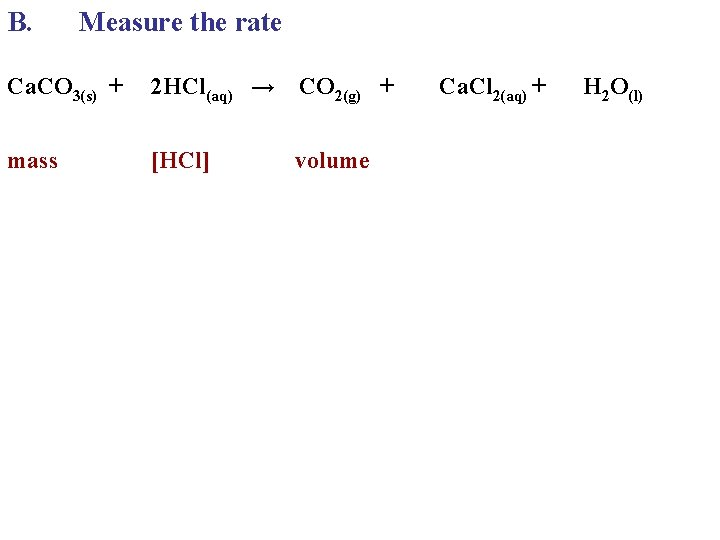B. Measure the rate Ca. CO 3(s) + 2 HCl(aq) → CO 2(g) + mass [HCl] volume Ca. Cl 2(aq) + H 2 O(l)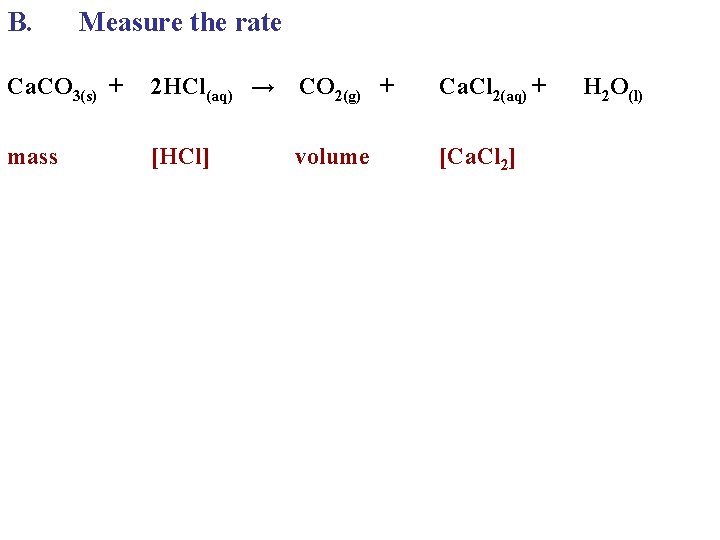B. Measure the rate Ca. CO 3(s) + 2 HCl(aq) → CO 2(g) + Ca. Cl 2(aq) + mass [Ca. Cl 2] [HCl] volume H 2 O(l)B. Measure the rate Ca. CO 3(s) + 2 HCl(aq) → CO 2(g) + Ca. Cl 2(aq) + H 2 O(l) mass [Ca. Cl 2] can’t [HCl] volumeB. Measure the rate Ca. CO 3(s) + 2 HCl(aq) → CO 2(g) + Ca. Cl 2(aq) + H 2 O(l) mass [Ca. Cl 2] can’t [HCl] volume over time Measure the decrease in mass of an open container Measure the increase in pressure of an closed container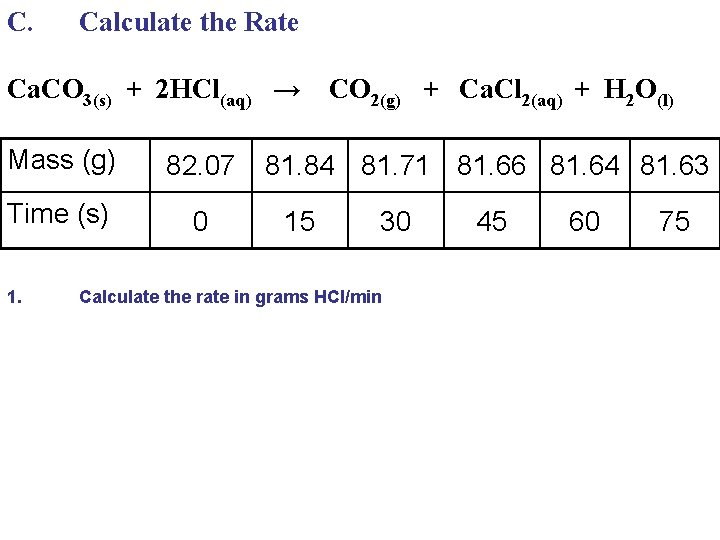C. Calculate the Rate Ca. CO 3(s) + 2 HCl(aq) → CO 2(g) + Ca. Cl 2(aq) + H 2 O(l) Mass (g) 82. 07 Time (s) 0 1. 81. 84 81. 71 81. 66 81. 64 81. 63 15 30 Calculate the rate in grams HCl/min 45 60 75C. Calculate the Rate Ca. CO 3(s) + 2 HCl(aq) → CO 2(g) + Ca. Cl 2(aq) + H 2 O(l) Mass (g) 82. 07 Time (s) 0 1. 81. 84 81. 71 81. 66 81. 64 81. 63 15 30 Calculate the rate in grams HCl/min 45 60 75C. Calculate the Rate Ca. CO 3(s) + 2 HCl(aq) → CO 2(g) + Ca. Cl 2(aq) + H 2 O(l) Mass (g) 82. 07 Time (s) 0 1. 81. 84 81. 71 81. 66 81. 64 81. 63 15 30 Calculate the rate in grams HCl/min (82. 07 - 81. 63) g CO 2 75 s 45 60 75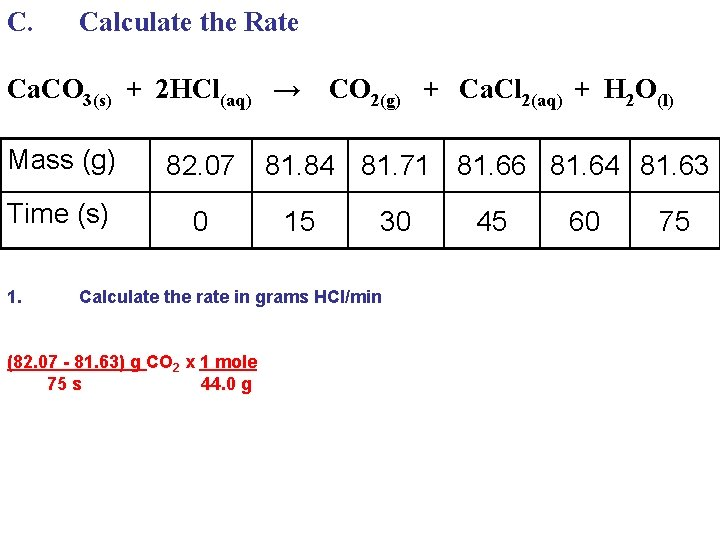C. Calculate the Rate Ca. CO 3(s) + 2 HCl(aq) → CO 2(g) + Ca. Cl 2(aq) + H 2 O(l) Mass (g) 82. 07 Time (s) 0 1. 81. 84 81. 71 81. 66 81. 64 81. 63 15 30 Calculate the rate in grams HCl/min (82. 07 - 81. 63) g CO 2 x 1 mole 75 s 44. 0 g 45 60 75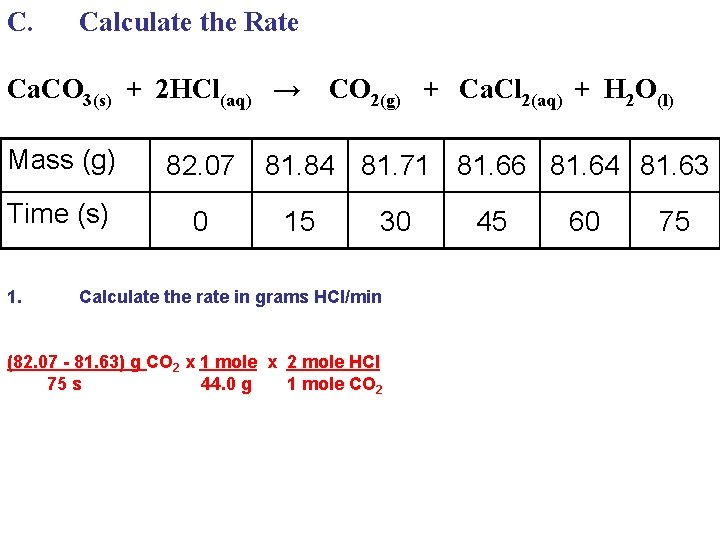C. Calculate the Rate Ca. CO 3(s) + 2 HCl(aq) → CO 2(g) + Ca. Cl 2(aq) + H 2 O(l) Mass (g) 82. 07 Time (s) 0 1. 81. 84 81. 71 81. 66 81. 64 81. 63 15 30 Calculate the rate in grams HCl/min (82. 07 - 81. 63) g CO 2 x 1 mole x 2 mole HCl 75 s 44. 0 g 1 mole CO 2 45 60 75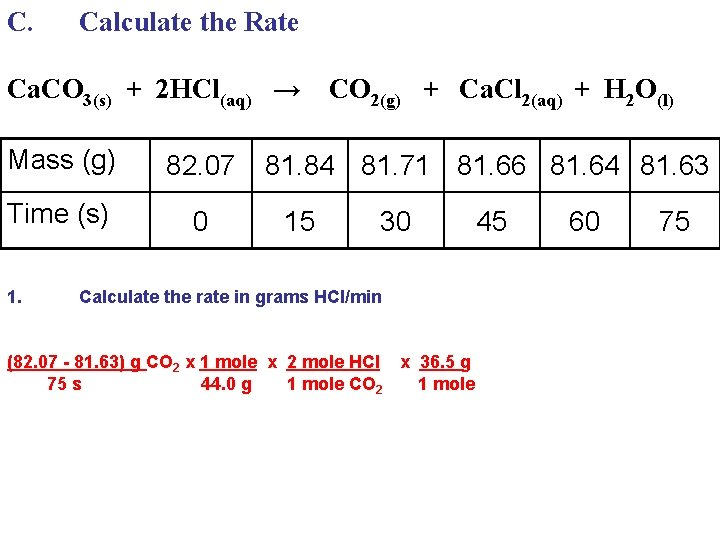C. Calculate the Rate Ca. CO 3(s) + 2 HCl(aq) → CO 2(g) + Ca. Cl 2(aq) + H 2 O(l) Mass (g) 82. 07 Time (s) 0 1. 81. 84 81. 71 81. 66 81. 64 81. 63 15 30 Calculate the rate in grams HCl/min (82. 07 - 81. 63) g CO 2 x 1 mole x 2 mole HCl x 36. 5 g 75 s 44. 0 g 1 mole CO 2 1 mole 45 60 75C. Calculate the Rate Ca. CO 3(s) + 2 HCl(aq) → CO 2(g) + Ca. Cl 2(aq) + H 2 O(l) Mass (g) 82. 07 Time (s) 0 1. 81. 84 81. 71 81. 66 81. 64 81. 63 15 30 45 60 75 Calculate the rate in grams HCl/min (82. 07 - 81. 63) g CO 2 x 1 mole x 2 mole HCl x 36. 5 g x 60 s = 0. 56 g/min 75 s 44. 0 g 1 mole CO 2 1 mole 1 minD. Collision Theory More Collisions Harder Collisions Lower Ea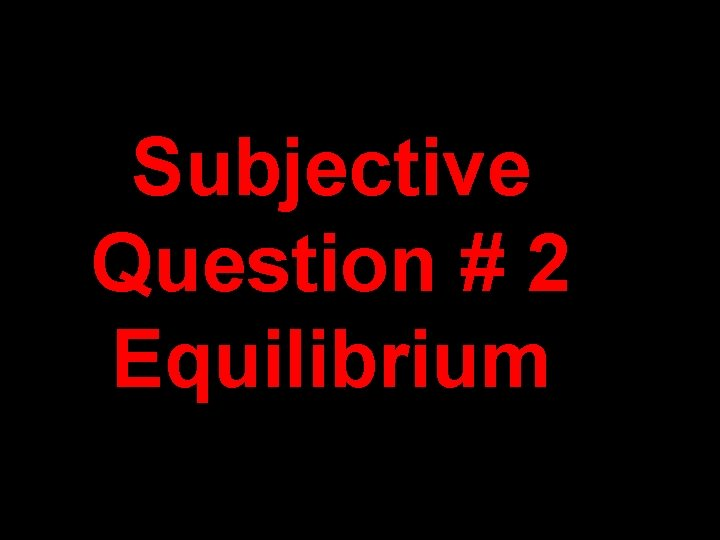Subjective Question # 2 EquilibriumWhen 0. 800 moles of SO 2 and 0. 800 moles of O 2 are placed into a 2. 00 litre container and allowed to reach equilibrium, the equilibrium [SO 3] is to be 0. 300 M. Calculate the Keq value. 2 SO 2 (g) + 1 O 2 (g) ⇋ 2 SO 3 (g)When 0. 800 moles of SO 2 and 0. 800 moles of O 2 are placed into a 2. 00 litre container and allowed to reach equilibrium, the equilibrium [SO 3] is to be 0. 300 M. Calculate the Keq value. 2 SO 2 (g) + 1 O 2 (g) ⇋ 2 SO 3 (g) I C E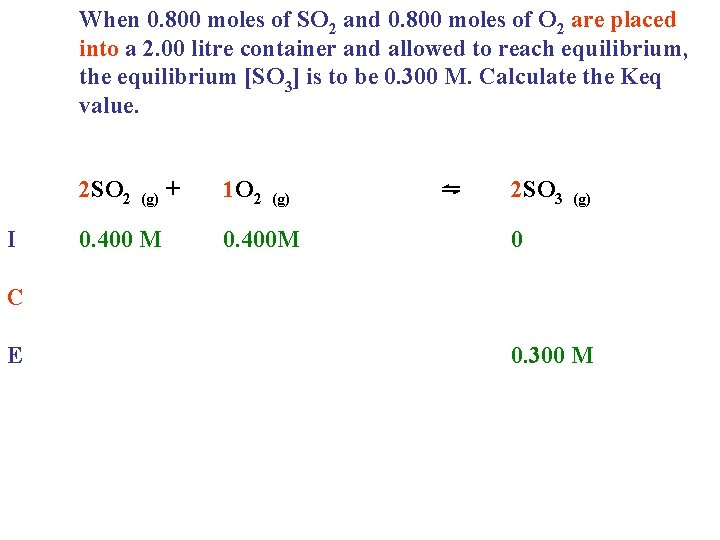When 0. 800 moles of SO 2 and 0. 800 moles of O 2 are placed into a 2. 00 litre container and allowed to reach equilibrium, the equilibrium [SO 3] is to be 0. 300 M. Calculate the Keq value. 2 SO 2 (g) + 1 O 2 (g) ⇋ 2 SO 3 (g) I 0. 400 M 0. 400 M 0 C E 0. 300 MWhen 0. 800 moles of SO 2 and 0. 800 moles of O 2 are placed into a 2. 00 litre container and allowed to reach equilibrium, the equilibrium [SO 3] is to be 0. 300 M. Calculate the Keq value. 2 SO 2 (g) + 1 O 2 (g) ⇋ 2 SO 3 (g) I 0. 400 M 0. 400 M C -0. 300 M -0. 150 M E 0 +0. 300 M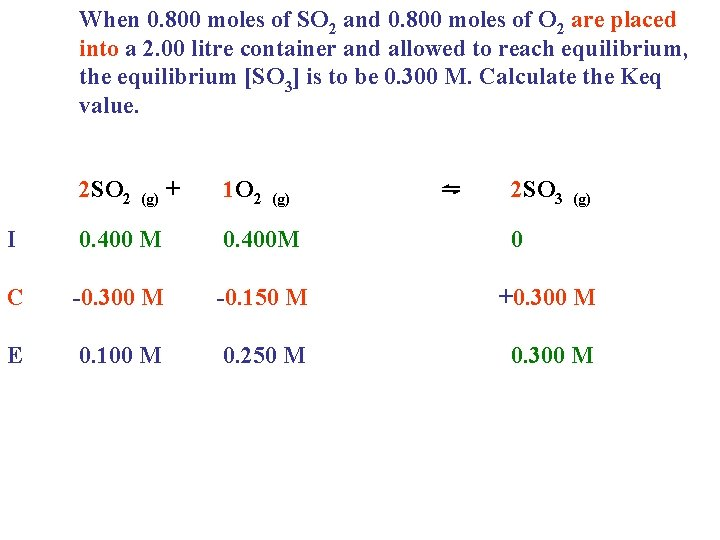When 0. 800 moles of SO 2 and 0. 800 moles of O 2 are placed into a 2. 00 litre container and allowed to reach equilibrium, the equilibrium [SO 3] is to be 0. 300 M. Calculate the Keq value. 2 SO 2 (g) + 1 O 2 (g) ⇋ 2 SO 3 (g) I 0. 400 M 0. 400 M C -0. 300 M -0. 150 M E 0. 100 M 0. 250 M 0 +0. 300 MWhen 0. 800 moles of SO 2 and 0. 800 moles of O 2 are placed into a 2. 00 litre container and allowed to reach equilibrium, the equilibrium [SO 3] is to be 0. 300 M. Calculate the Keq value. 2 SO 2 (g) + 1 O 2 (g) ⇋ 2 SO 3 (g) I 0. 400 M 0. 400 M C -0. 300 M -0. 150 M E 0. 100 M 0. 250 M 0 +0. 300 M Equilibrium concentrations go in the equilibrium equation! Keq = [SO 3]2 [SO ]2[O ]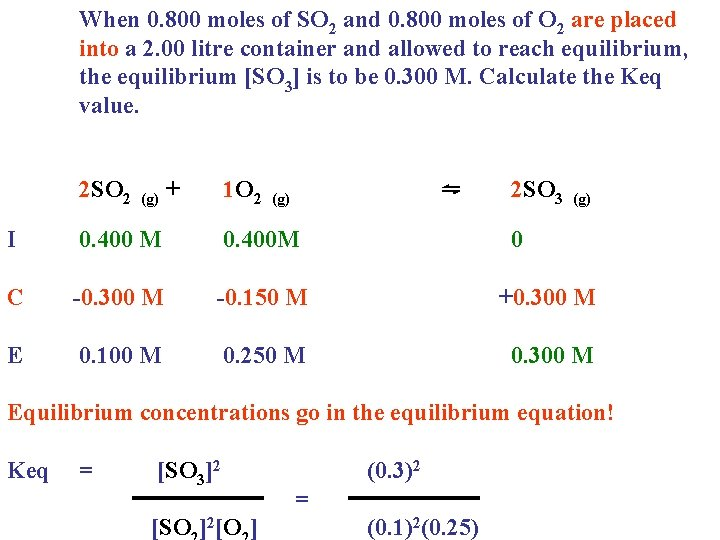When 0. 800 moles of SO 2 and 0. 800 moles of O 2 are placed into a 2. 00 litre container and allowed to reach equilibrium, the equilibrium [SO 3] is to be 0. 300 M. Calculate the Keq value. 2 SO 2 (g) + 1 O 2 (g) ⇋ 2 SO 3 (g) I 0. 400 M 0. 400 M 0 C -0. 300 M -0. 150 M E 0. 100 M +0. 300 M 0. 250 M 0. 300 M Equilibrium concentrations go in the equilibrium equation! Keq = [SO 3]2 [SO ]2[O ] (0. 3)2 = (0. 1)2(0. 25)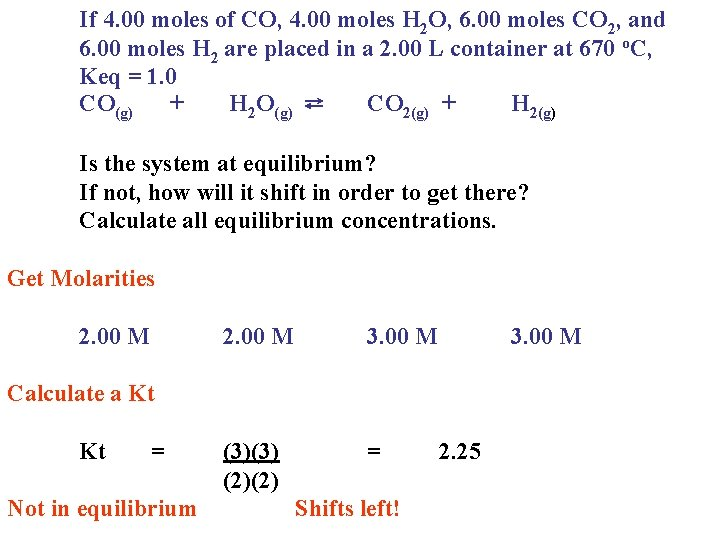If 4. 00 moles of CO, 4. 00 moles H 2 O, 6. 00 moles CO 2, and 6. 00 moles H 2 are placed in a 2. 00 L container at 670 o. C, Keq = 1. 0 CO(g) + H 2 O(g) ⇄ CO 2(g) + H 2(g) Is the system at equilibrium? If not, how will it shift in order to get there? Calculate all equilibrium concentrations. Get Molarities 2. 00 M 3. 00 M (3)(3) (2)(2) = 3. 00 M Calculate a Kt Kt = Not in equilibrium Shifts left! 2. 25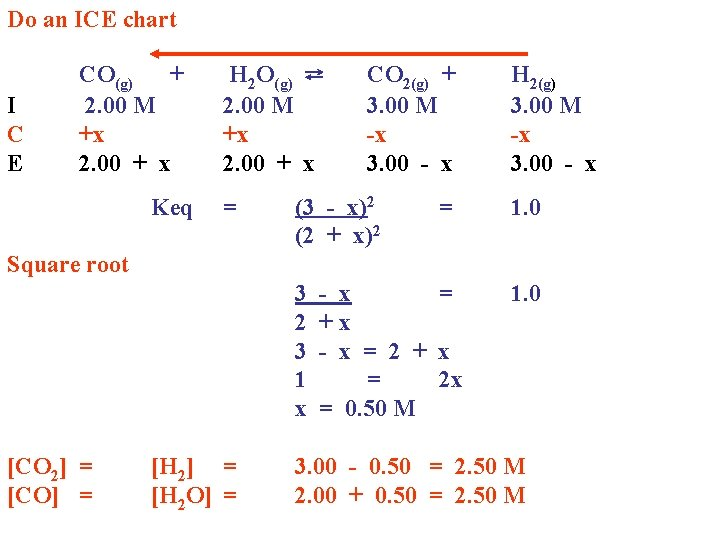Do an ICE chart I C E CO(g) + 2. 00 M +x 2. 00 + x Keq H 2 O(g) ⇄ 2. 00 M +x 2. 00 + x = CO 2(g) + 3. 00 M -x 3. 00 - x (3 - x)2 (2 + x)2 = H 2(g) 3. 00 M -x 3. 00 - x 1. 0 Square root 3 - x = 2 + x 3 - x = 2 + x 1 = 2 x x = 0. 50 M [CO 2] = [CO] = [H 2 O] = 1. 0 3. 00 - 0. 50 = 2. 50 M 2. 00 + 0. 50 = 2. 50 MSubjective Question # 3 Solubility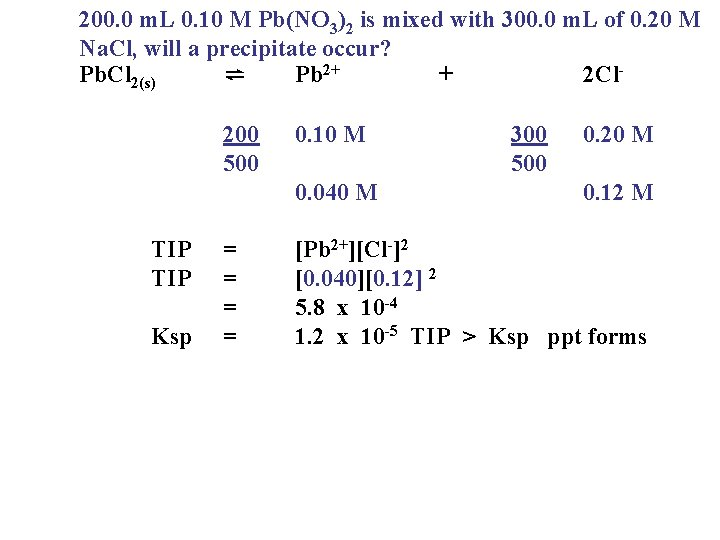200. 0 m. L 0. 10 M Pb(NO 3)2 is mixed with 300. 0 m. L of 0. 20 M Na. Cl, will a precipitate occur? Pb. Cl 2(s) ⇌ Pb 2+ + 2 Cl 200 500 0. 10 M 0. 040 M TIP Ksp = = 300 500 0. 20 M 0. 12 M [Pb 2+][Cl-]2 [0. 040][0. 12] 2 5. 8 x 10 -4 1. 2 x 10 -5 TIP > Ksp ppt formsCalculate the maximum number of grams Ba. Cl 2 that will dissolve in 0. 50 L of 0. 20 M Ag. NO 3 solution. Ag. Cl(s) ⇄ Ag+ + 0. 20 M Ksp = [Ag+][Cl-] 1. 8 x 10 -10 = [Cl-] = Ba. Cl 2(s) 4. 5 x 10 -10 M ⇄ Cl- [0. 20][Cl-] 9. 0 x 10 -10 M Ba 2+ + 2 Cl 9. 0 x 10 -10 M 0. 50 L x 4. 5 x 10 -10 mole x 208. 3 g = 4. 7 x 10 -8 g 1 L molePb. Cl 2(s) ⇌ Pb 2+ Ksp = 4 s 3 + 2 Cl-Subjective Question # 4 to 6 Acids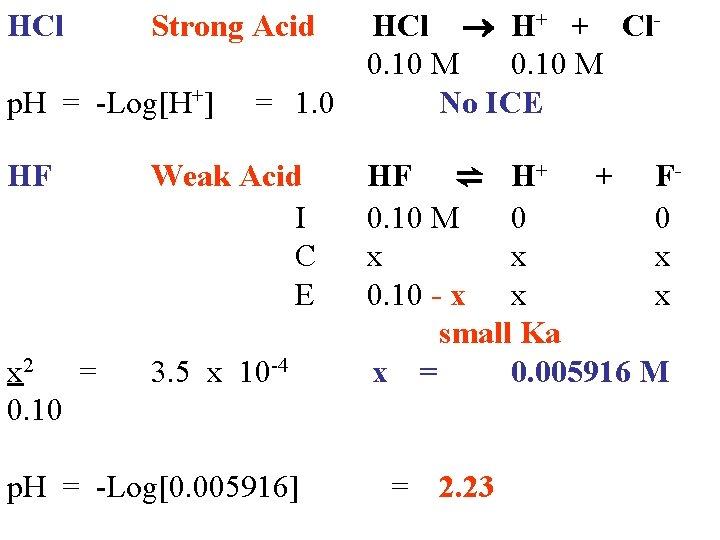HCl Strong Acid HCl H+ + Cl 0. 10 M p. H = -Log[H+] = 1. 0 No ICE HF x 2 = 0. 10 Weak Acid HF ⇌ H+ + FI 0. 10 M 0 0 C x x x E 0. 10 - x x x small Ka x = 3. 5 x 10 -4 0. 005916 M p. H = -Log[0. 005916] = 2. 23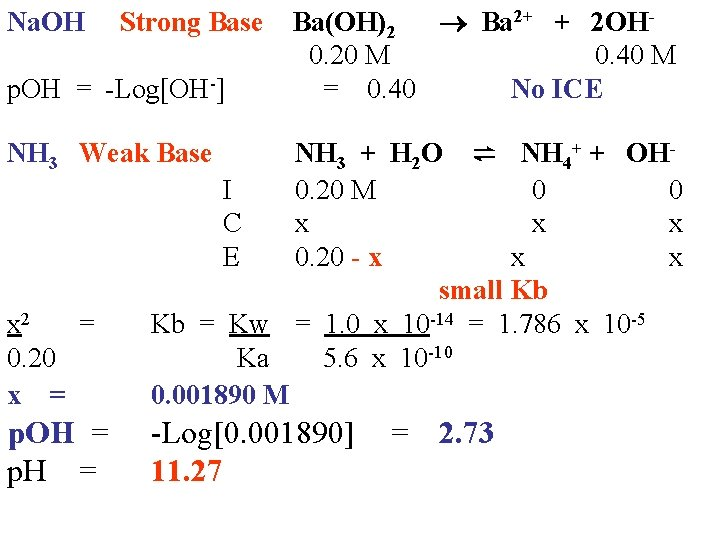Na. OH Strong Base Ba(OH)2 Ba 2+ + 2 OH 0. 20 M 0. 40 M p. OH = -Log[OH-] = 0. 40 No ICE NH 3 Weak Base I C E x 2 = 0. 20 x = NH 3 + H 2 O ⇌ NH 4+ + OH 0. 20 M 0 0 x x x 0. 20 - x x x small Kb Kb = Kw = 1. 0 x 10 -14 = 1. 786 x 10 -5 Ka 5. 6 x 10 -10 0. 001890 M p. OH = -Log[0. 001890] = 2. 73 p. H = 11. 27Subjective Question 7 & 8 RedoxReview of Cells Electrochemical Is a power supply Spontaneous (+ ve) Makes electricity Reduction is highest on Chart Electrolytic Requires power supply Nonspontaneous(-ve) Makes chemicals Reduction is the –veFor all cells: Cations migrate to the cathode, which is the site of reduction. Anions migrate to the anode, which is the site of oxidation. Electrons travel through the wire from anode to cathode.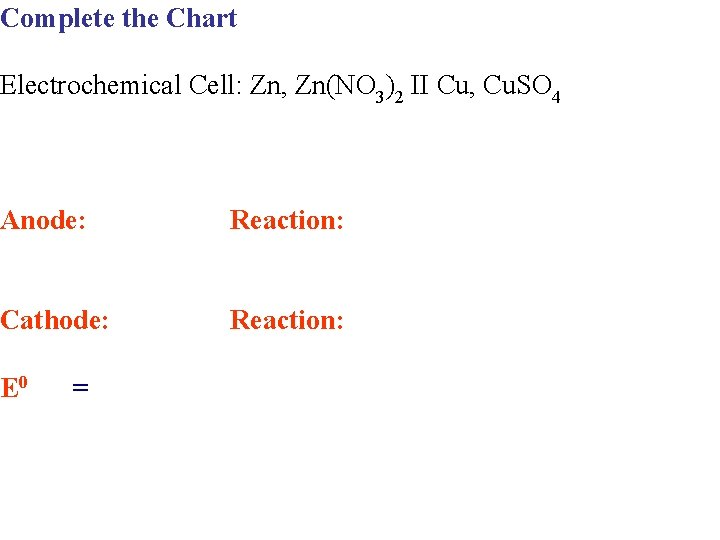Complete the Chart Electrochemical Cell: Zn, Zn(NO 3)2 II Cu, Cu. SO 4 Anode: Reaction: Cathode: Reaction: E 0 =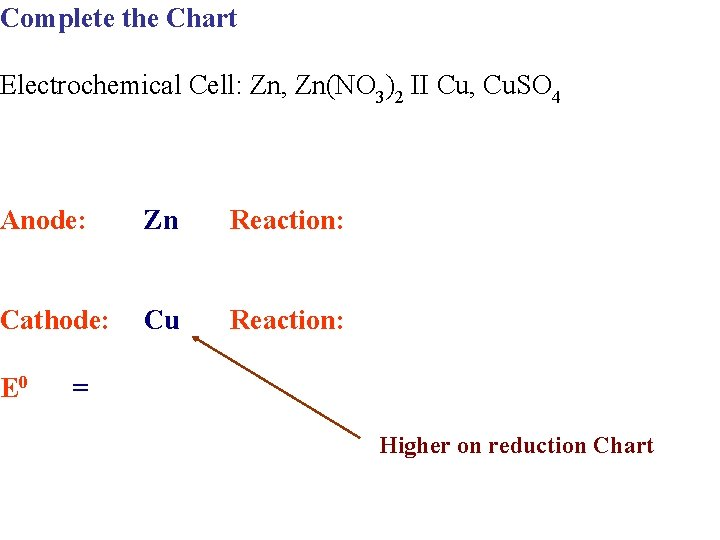Complete the Chart Electrochemical Cell: Zn, Zn(NO 3)2 II Cu, Cu. SO 4 Anode: Zn Reaction: Cathode: Cu Reaction: E 0 = Higher on reduction ChartComplete the Chart Electrochemical Cell: Zn, Zn(NO 3)2 II Cu, Cu. SO 4 Anode: Zn Reaction: Zn → Zn 2+ + 2 e- 0. 76 v Cathode: Cu Reaction: E 0 = Higher on reduction Chart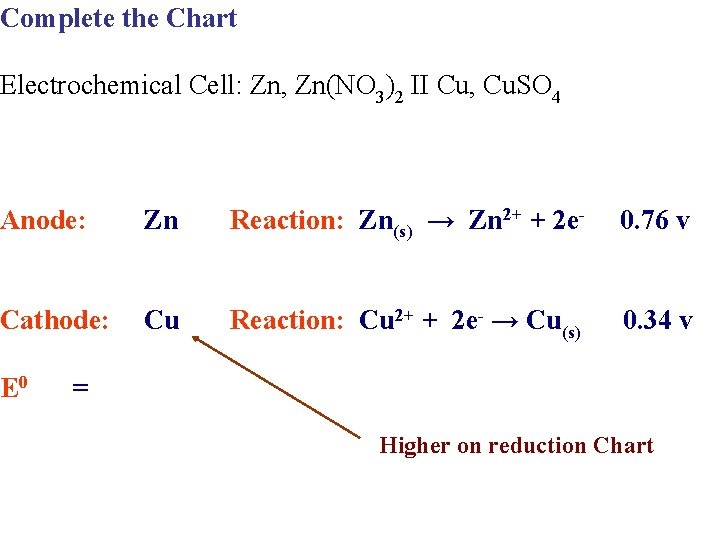Complete the Chart Electrochemical Cell: Zn, Zn(NO 3)2 II Cu, Cu. SO 4 Anode: Zn Reaction: Zn(s) → Zn 2+ + 2 e- 0. 76 v Cathode: Cu Reaction: Cu 2+ + 2 e- → Cu(s) 0. 34 v E 0 = Higher on reduction ChartComplete the Chart Electrochemical Cell: Zn, Zn(NO 3)2 II Cu, Cu. SO 4 Anode: Zn Reaction: Zn(s) → Zn 2+ + 2 e- 0. 76 v Cathode: Cu Reaction: Cu 2+ + 2 e- → Cu(s) 0. 34 v E 0 1. 10 v = Higher on reduction Chart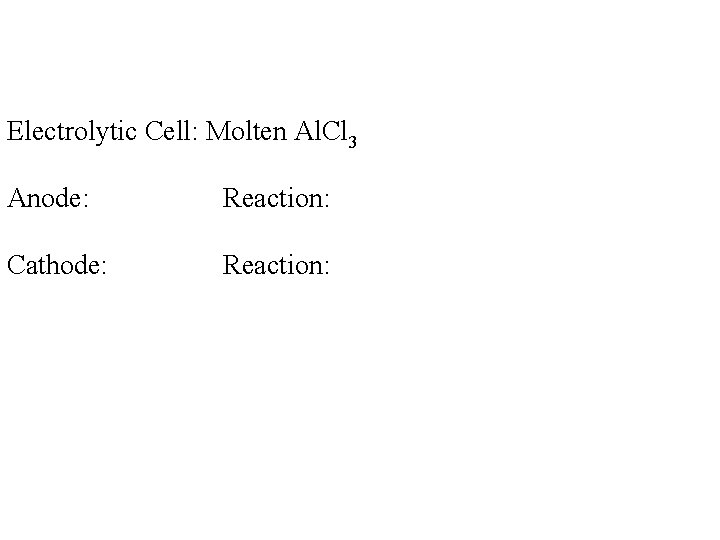Electrolytic Cell: Molten Al. Cl 3 Anode: Reaction: Cathode: Reaction: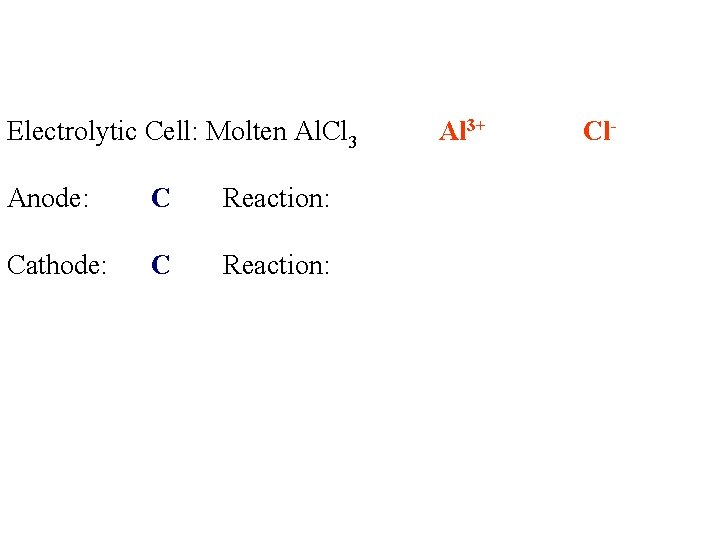Electrolytic Cell: Molten Al. Cl 3 Anode: C Reaction: Cathode: C Reaction: Al 3+ Cl-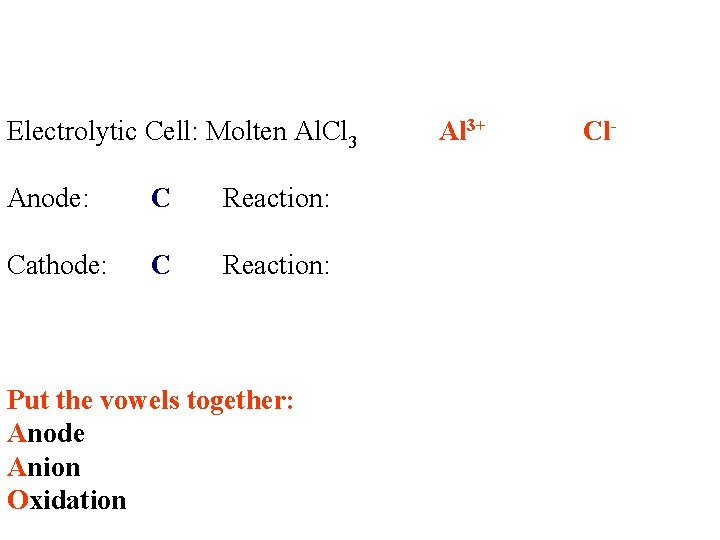Electrolytic Cell: Molten Al. Cl 3 Anode: C Reaction: Cathode: C Reaction: Put the vowels together: Anode Anion Oxidation Al 3+ Cl-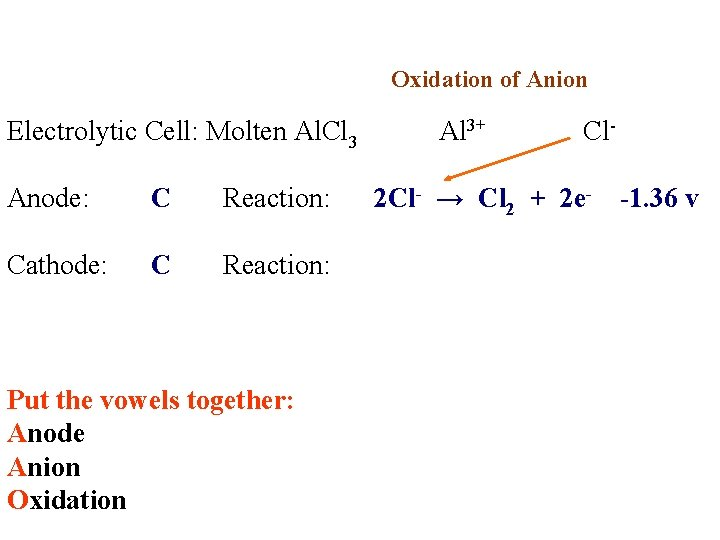Oxidation of Anion Electrolytic Cell: Molten Al. Cl 3 Al 3+ Cl Anode: C Reaction: 2 Cl- → Cl 2 + 2 e- -1. 36 v Cathode: C Reaction: Put the vowels together: Anode Anion Oxidation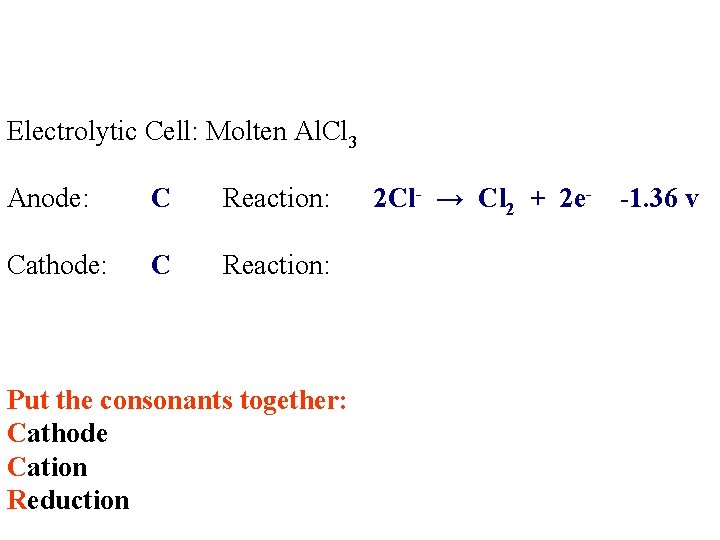Electrolytic Cell: Molten Al. Cl 3 Anode: C Reaction: 2 Cl- → Cl 2 + 2 e- -1. 36 v Cathode: C Reaction: Put the consonants together: Cathode Cation ReductionElectrolytic Cell: Molten Al. Cl 3 Anode: C Reaction: 2 Cl- → Cl 2 + 2 e- -1. 36 v Cathode: C Reaction: Al 3+ + 3 e- → Al -1. 66 v Put the consonants together: Cathode Cation Reduction of CationElectrolytic Cell: Molten Al. Cl 3 Anode: C Reaction: 2 Cl- → Cl 2 + 2 e- -1. 36 v Cathode: C Reaction: Al 3+ + 3 e- → Al -1. 66 v E 0 = -3. 02 v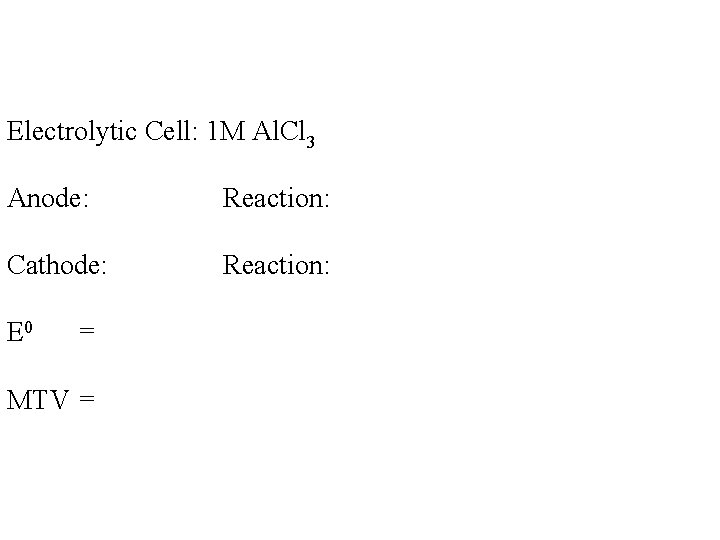Electrolytic Cell: 1 M Al. Cl 3 Anode: Reaction: Cathode: Reaction: E 0 = MTV =Electrolytic Cell: 1 M Al. Cl 3 Anode: C Reaction: Cathode: C Reaction: E 0 = MTV = H 2 O → 1/2 O 2 + 2 H+ + 2 e-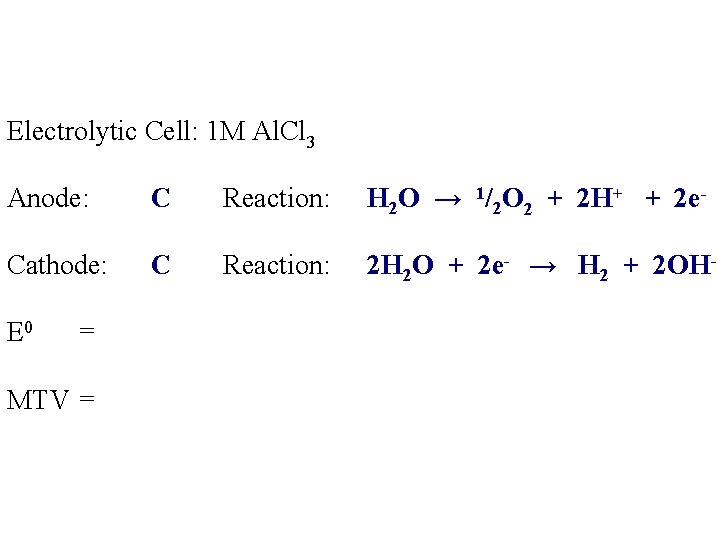Electrolytic Cell: 1 M Al. Cl 3 Anode: C Reaction: Cathode: C Reaction: E 0 = MTV = H 2 O → 1/2 O 2 + 2 H+ + 2 e 2 H 2 O + 2 e- → H 2 + 2 OH-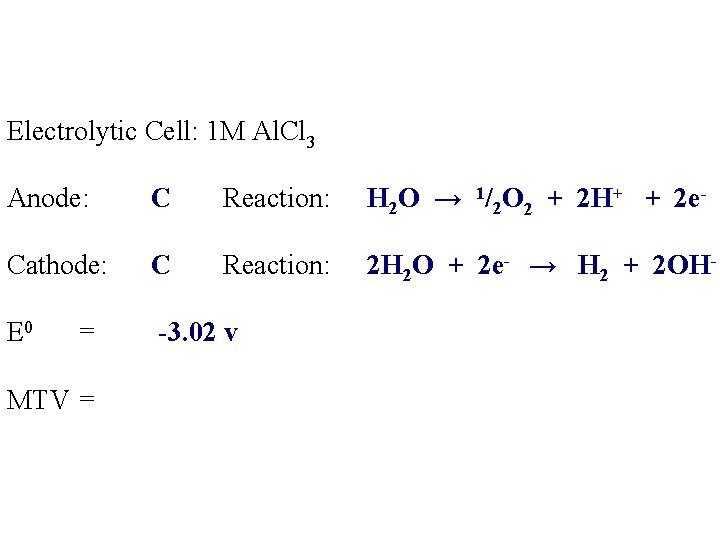Electrolytic Cell: 1 M Al. Cl 3 Anode: C Reaction: Cathode: C Reaction: E 0 = -3. 02 v MTV = H 2 O → 1/2 O 2 + 2 H+ + 2 e 2 H 2 O + 2 e- → H 2 + 2 OH-Electrolytic Cell: 1 M Al. Cl 3 Anode: C Reaction: Cathode: C Reaction: E 0 = -3. 02 v MTV = +3. 02 v H 2 O → 1/2 O 2 + 2 H+ + 2 e 2 H 2 O + 2 e- → H 2 + 2 OH-